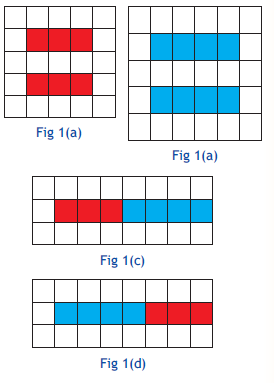# CBSE Class 6 Maths Activity 1

Read and download CBSE Class 6 Maths Activity 1 chapter in NCERT book for Class 6 Mathematics. You can download latest NCERT eBooks for 2022 chapter wise in PDF format free from Studiestoday.com. This Mathematics textbook for Class 6 is designed by NCERT and is very useful for students. Please also refer to the NCERT solutions for Class 6 Mathematics to understand the answers of the exercise questions given at the end of this chapter

## Activity 1 Class 6 Mathematics NCERT

Class 6 Mathematics students should refer to the following NCERT Book chapter Activity 1 in standard 6. This NCERT Book for Grade 6 Mathematics will be very useful for exams and help you to score good marks

### Activity 1 NCERT Class 6

(a) To verify that addition is commutative for whole numbers, by paper cutting and pasting.

(b) To verify that multiplication is commutative for whole numbers, by paper cutting and pasting

Learning Objective : To understand that addition as well as multiplication are commutative for whole numbers.

Pre requisite : (i) Knowledge of addition and multiplication of whole numbers.

(ii) Knowledge of commutative property of whole numbers.

Materials required : Grid papers, a pair of scissors and glue

Procedure (a) : (a) To verify 3 + 4 = 4 + 3

Step 1. Cut out two strips each of length 3 units from a grid paper and shade them with any colour (say Red) Fig. 1(a).

Step 2. Cut two strips each of length 4 units from a grid paper and shade them with different colour (say Blue ) Fig. 1(b).

Step 3. For representing 3 + 4, paste one strip of length 3 units and then paste another strip of length 4 units in the same line on the grid paper without

leaving any gap Fig. 1(c).

Step 4. For representing 4 + 3, paste one strip of length 4 units and then paste another strip of length 3 units in the same on the same grid paper line

without leaving any gap Fig. 1(d).

Step 5. Now, compare (by counting number of boxes) the length of strips obtained in Step 3 and Step 4.

Step 6. Repeat the activity for some more pairs of whole numbers and write your result.

Observations:

Number of shaded boxes in the presentation of 3 + 4 = …………….

Number of shaded boxes in the presentation of 4 + 3 = …………….Please refer to the link below - CBSE Class 6 Maths Activity 1

 NCERT Class 6 Maths Knowing our Numbers
 NCERT Class 6 Maths Mensuration
 NCERT Class 6 Maths Algebra
 NCERT Class 6 Maths Ratio and Proportion
 NCERT Class 6 Maths Symmetry
 NCERT Class 6 Maths Practical Geometry
 NCERT Class 6 Maths Whole Numbers
 NCERT Class 6 Maths Playing with Numbers
 NCERT Class 6 Maths Basic Geometrical Ideas
 NCERT Class 6 Maths Understanding Elementary Shapes
 NCERT Class 6 Maths Integers
 NCERT Class 6 Maths Fractions
 NCERT Class 6 Maths Decimals
 NCERT Class 6 Maths Data Handling
 NCERT Class 6 Maths Answers to all Chapters
 NCERT Class 6 Maths Brain Teasers